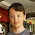## Friday, August 7, 2015

### Optimizing Part of the Objective Function

A somewhat curious question showed up on a forum today. The author of the question has an optimization model (I'll assume it is either a linear program or mixed integer linear program) of the form \begin{alignat*}{2} & \textrm{maximize} & & \sum_{i=1}^{N}x_{i}\\ & \textrm{s.t.} & & x\in\mathcal{X} \end{alignat*} where the feasible region $\mathcal{X}$ is presumably polyhedral. What the author wants to do is instead maximize the sum of the $K$ largest terms in the objective, for some fixed $K<N$. The question was how to do this.

In effect, the author wants to selectively turn some terms on and others off in the objective function. Any time I think about turning things on and off, I immediately think of using binary variables as the "switches". That in turn suggests the likely need for auxiliary variables and the very likely need for a priori bounds on the things being turned on and off. Here is one solution, step by step, assuming that the $x$ variables are nonnegative and that we know a finite upper bound $U_i$ for each $x_i$.

#### 1. Introduce a binary variable for each term to be switched on/off.

So we add variables $z_i \in \{0,1\}$ for $i\in 1\dots N$, with $z_i=1$ if and only if $x_i$ is to be counted.

#### 2. Limit the number of terms to count.

This is just the constraint $$\sum_{i=1}^N z_i = K$$ (with the option to change the equality to $\le$ if you want up to $K$ terms counted.

#### 3. Replace the objective terms with surrogates that can be turned on/off.

We will add real variables $y_1,\dots,y_N$ and make the objective $$\textrm{maximize} \sum_{i=1}^N y_i.$$

#### 4. Connect the surrogate variables to the original variables and the on-off decisions.

Here we benefit from a key property: if we limit the objective function to $K$ terms, the fact that we are maximizing will naturally favor the $K$ largest terms. So we just need the following constraints: \begin{alignat*}{2} y_{i} & \le x_{i} & & \forall i\in\left\{ 1,\dots,N\right\} \\ y_{i} & \le U_{i}z_{i} & \quad & \forall i\in\left\{ 1,\dots,N\right\} . \end{alignat*}If $z_i = 0$, the second constraint will force $y_i=0$ and the term will not contribute to the objective function. If $z_i=1$, the second constraint will become vacuous and the first term will allow $y_i$ to contribute an amount up to $x_i$ to the objective. Since the objective is being maximized, $y_i=x_i$ is certain to occur.

A symmetric version of this will work to minimize the sum of the $K$ smallest terms in the objective. Minimizing the sum of the largest terms or maximizing the sum of the smallest terms is a bit trickier, requiring some extra constraints to enforce $y_i=x_i$ when $z_i = 1$. Oops! It's actually easier, as pointed out in a comment in my next post.

1.Interesting post, but the formulas are not rendering (at least in my browser).

2.The formulas are also not rending on my browser.

3.It seems MathJax.org switched their content distribution network without my knowing it. For some unfathomable reason, when I preview my posts in Blogger (with the expired CDN URL), they still render properly. I just fixed this post. Hopefully the math now renders (?).

1.Yes, looks fine now.

2.Thanks for letting me know.

Due to recent spamming, comments are being moderated. If this is your first time commenting on the blog, please read the Ground Rules for Comments. In particular, if you want to ask an operations research-related question not relevant to this post, consider asking it on Mathematics Stack Exchange.